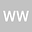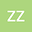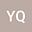Ideal thermodynamic efficiency equation for continuous distillation of ideal binary mixture
•••• +2
• Weiguo Wang,
• Zhen Zeng,
• Yuan-Hang Qin,
• Tielin Wang,
• Cunwen Wang
Weiguo Wang
Wuhan Institute of Technology
Author ProfileZhen Zeng
Wuhan Institute of Technology
Author ProfileYuan-Hang Qin
Wuhan Institute of Technology
Author ProfileTielin Wang
Wuhan Institute of Technology
Author ProfileCunwen Wang
Wuhan Institute of Technology
Author Profile## Abstract

Determining the conversion efficiency of thermal exergy into the work of separation is of great importance for distillation process. In this study, an ideal thermodynamic efficiency equation not containing physical property data was proposed for calculating the thermodynamic efficiency of the continuous distillation process of ideal binary mixture. Benzene-toluene mixture was taken as an ideal binary mixture, and the accurate and approximate thermodynamic efficiencies of the continuous distillation of benzene-toluene ideal mixture were calculated by the thermodynamic efficiency equation based on the relevant physical property data and the proposed ideal thermodynamic efficiency equation, respectively. The results showed that the difference between the calculated thermodynamic efficiencies was less than 5%, demonstrating the feasibility of calculating the thermodynamic efficiency of the continuous distillation process of ideal binary mixture using the ideal thermodynamic efficiency equation.